# Quick Tour¶

## Set up¶

### Interactive Rings shell¶

To taste what Rings can do, one can try interactive Rings session with Ammonite REPL. One can install Rings.repl with Homebrew:

$brew install PoslavskySV/rings/rings.repl  or just by typing the following commands at the prompt: $ sudo sh -c '(echo "#!/usr/bin/env sh" && curl -L https://github.com/lihaoyi/Ammonite/releases/download/1.1.2/2.12-1.1.2) > /usr/local/bin/amm && chmod +x /usr/local/bin/amm'
$sudo sh -c 'curl -L -o /usr/local/bin/rings.repl https://git.io/vd7EY && chmod +x /usr/local/bin/rings.repl'  Now run Rings.repl: $ rings.repl
Rings 2.5.7: efficient Java/Scala library for polynomial rings

@ implicit val ring = MultivariateRing(Z, Array("x", "y", "z"))
ring: MultivariateRing[IntZ] = MultivariateRing(Z, Array("x", "y", "z"), LEX)

@ val poly1 = ring("x + y - z").pow(8)
poly1: MultivariatePolynomial[IntZ] = z^8-8*y*z^7+28*y^2*z^6-56*y^3*z^5+70*y^4*z^4-56...

@ val poly2 = ring("x - y + z").pow(8)
poly1: MultivariatePolynomial[IntZ] = z^8-8*y*z^7+28*y^2*z^6-56*y^3*z^5+70*y^4*z^4-56...

@ Factor(poly1 - poly2)
res13: FactorDecomposition[MultivariatePolynomial[IntZ]] = 16*x*(-z+y)*(z^2-2*y*z+y^2+x^2)*(z^4-4*y*z^3+6*y^2*z^2-4*y^3*z+y^4+6*x^2*z^2-12*x^2*y*z+6*x^2*y^2+x^4)


### Java/Scala library¶

Rings is currently available for Java and Scala. To get started with Scala SBT, simply add the following dependence to your build.sbt file:

libraryDependencies += "cc.redberry" %% "rings.scaladsl" % "2.5.7"


For using Rings solely in Java there is Maven artifact:

<dependency>
<groupId>cc.redberry</groupId>
<artifactId>rings</artifactId>
<version>2.5.7</version>
</dependency>


## Examples: rings, ideals, Gröbner bases, GCDs & factorization¶

Below examples can be evaluated directly in the Rings.repl. For Scala/Java the following preambula will import all required things from Rings library:

import cc.redberry.rings

import rings.poly.PolynomialMethods._
import syntax._

import cc.redberry.rings.*;
import cc.redberry.rings.poly.*;
import cc.redberry.rings.poly.univar.*;
import cc.redberry.rings.poly.multivar.*;
import cc.redberry.rings.bigint.BigInteger;

import static cc.redberry.rings.poly.PolynomialMethods.*;
import static cc.redberry.rings.Rings.*;


### Some built-in rings¶

Polynomial rings over $$Z$$ and $$Q$$:

// Ring Z[x]
UnivariateRing(Z, "x")
// Ring Z[x, y, z]
MultivariateRing(Z, Array("x", "y", "z"))
// Ring Q[a, b, c]
MultivariateRing(Q, Array("a", "b", "c"))

// Ring Z[x]
UnivariateRing(Z);
// Ring Z[x, y, z]
MultivariateRing(3, Z);
// Ring Q[x, y, z]
MultivariateRing(3, Q);


Polynomial rings over $$Z_p$$:

// Ring Z/3[x]
UnivariateRingZp64(3, "x")
// Ring Z/3[x, y, z]
MultivariateRingZp64(3, Array("x", "y", "z"))
// Ring Z/p[x, y, z] with p = 2^107 - 1 (Mersenne prime)
MultivariateRing(Zp(Z(2).pow(107) - 1), Array("x", "y", "z"))

// Ring Z/3[x]
UnivariateRingZp64(3);
// Ring Z/3[x, y, z]
MultivariateRingZp64(3, 3);
// Ring Z/p[x, y, z] with p = 2^107 - 1 (Mersenne prime)
MultivariateRing(3, Zp(BigInteger.ONE.shiftLeft(107).decrement()));


Galois fields:

// Galois field with cardinality 7^10
// (irreducible polynomial will be generated automatically)
GF(7, 10, "x")
// GF(7^3) generated by irreducible polynomial "1 + 3*z + z^2 + z^3"
GF(UnivariateRingZp64(7, "z")("1 + 3*z + z^2 + z^3"), "z")

// Galois field with cardinality 7^10
// (irreducible polynomial will be generated automatically)
GF(7, 10);
// GF(7^3) generated by irreducible polynomial "1 + 3*z + z^2 + z^3"
GF(UnivariatePolynomialZ64.create(1, 3, 1, 1).modulus(7));


Fields of rational functions:

// Field of fractions of univariate polynomials Z[x]
Frac(UnivariateRing(Z, "x"))
// Field of fractions of multivariate polynomials Z/19[x, y, z]
Frac(MultivariateRingZp64(19, Array("x", "y", "z")))

// Field of fractions of univariate polynomials Z[a]
Frac(UnivariateRing(Z));
// Field of fractions of multivariate polynomials Z/19[a, b, c]
Frac(MultivariateRingZp64(3, 19));


### Univariate polynomials¶

Some algebra in Galois field $$GF(17,9)$$:

// Galois field GF(17, 9) with irreducible
// poly in Z/17[t] generated automaticaly
implicit val ring = GF(17, 9, "t")

// pick some random field element
val a = ring.randomElement()
// raise field element to the power of 1000
val b = a.pow(1000)
// reciprocal of field element
val c = 1 / b

assert ( b * c === 1)

// explicitly parse field element from string:
// input poly will be automatically converted to
// element of GF(17, 9) (reduced modulo field generator)
val d = ring("1 + t + t^2 + t^3 + 15 * t^999")
// do some arbitrary math ops in the field
val some = a / (b + c) + a.pow(6) - a * b * c * d

// Galois field GF(17, 9) with irreducible
// poly in Z/17[t] generated automaticaly
FiniteField<UnivariatePolynomialZp64> ring = GF(17, 9);

// pick some random field element
UnivariatePolynomialZp64 a = ring.randomElement();
// raise field element to the power of 1000
UnivariatePolynomialZp64 b = ring.pow(a, 1000);
// reciprocal of field element
UnivariatePolynomialZp64 c = ring.reciprocal(b);

assert ring.multiply(b, c).isOne();

// explicitly parse field element from string:
// input poly will be automatically converted to
// element of GF(17, 9) (reduced modulo field generator)
UnivariatePolynomialZp64 d = ring.parse("1 + x + x^2 + x^3 + 15*x^999");
// do some arbitrary math ops in the field
ring.pow(a, 6),
ring.negate(ring.multiply(a, b, c, d)));


Extended GCD in $$Z_{17}[x]$$:

// polynomial ring Z/17[x]
implicit val ring = UnivariateRingZp64(17, "x")
// parse ring element
val x = ring("x")

// construct some polynomials
val poly1 = 1 + x + x.pow(2) + x.pow(3)
val poly2 = 1 + 2 * x + 9 * x.pow(2)

// compute (gcd, s, t) such that s * poly1 + t * poly2 = gcd
val Array(gcd, s, t) = PolynomialExtendedGCD(poly1, poly2)
assert (s * poly1 + t * poly2 == gcd)

println((gcd, s, t))

// construct polynomials in Z/17[x] given by arrays of coefficients
UnivariatePolynomialZp64
a = UnivariatePolynomialZ64.create(1, 1, 1, 1).modulus(17),
b = UnivariatePolynomialZ64.create(1, 2, 9).modulus(17);

// compute xgcd array such that xgcd * poly1 + xgcd * poly2 = xgcd
UnivariatePolynomialZp64[] xgcd = PolynomialExtendedGCD(a, b);

System.out.println(Arrays.toString(xgcd));


Factor polynomial in $$Z_{17}[x]$$:

// polynomial ring Z/17[x]
implicit val ring = UnivariateRingZp64(17, "x")x

// parse polynomial from string
val poly = ring("4 + 8*x + 12*x^2 + 5*x^5 - x^6 + 10*x^7 + x^8")

// factorize poly
val factors = Factor(poly)
println(factors)

// the modulus
long modulus = 17;
// parse univariate poly over Z/17 from string
UnivariatePolynomialZp64 poly = UnivariatePolynomialZp64
.parse("4 + 8*x + 12*x^2 + 5*x^5 - x^6 + 10*x^7 + x^8", modulus);

// factorize poly
FactorDecomposition<UnivariatePolynomialZp64> factors = Factor(poly);
System.out.println(factors);


Coefficient rings with arbitrary large characteristic are available:

// coefficient ring Z/1237940039285380274899124357 (the next prime to 2^100)
val modulus = Z("1267650600228229401496703205653")
val cfRing  = Zp(modulus)

// ring Z/1237940039285380274899124357[x]
implicit val ring = UnivariateRing(cfRing, "x")
val poly = ring("4 + 8*x + 12*x^2 + 5*x^5 + 16*x^6 + 27*x^7 + 18*x^8")

// factorize poly
println(Factor(poly))

// coefficient ring Z/1237940039285380274899124357 (the next prime to 2^100)
IntegersZp cfRing = Zp(new BigInteger("1267650600228229401496703205653"));

UnivariatePolynomial<BigInteger> poly = UnivariatePolynomial
.parse("4 + 8*x + 12*x^2 + 5*x^5 - x^6 + 10*x^7 + x^8", cfRing);

// factorize poly
System.out.println(Factor(poly));


(large primes can be generated with BigPrimes.nextPrime method, see Prime numbers).

Ring of univariate polynomials over elements of Galois field $$GF(7,3)[x]$$:

// elements of coefficient field GF(7,3) are represented as polynomials
// over "z" modulo irreducible polynomial "1 + 3*z + z^2 + z^3"
val cfRing = GF(UnivariateRingZp64(7, "z")("1 + 3*z + z^2 + z^3"), "z")

assert(cfRing.characteristic().intValue() == 7)
assert(cfRing.cardinality().intValue() == 343)

// polynomial ring GF(7^3)[x]
implicit val ring = UnivariateRing(cfRing, "x")

// parse poly in GF(7^3)[x] from string
// coefficients of polynomials in GF(7,3)[x] are elements
// of GF(7,3) that is polynomials over "z"
val poly = ring("1 - (1 - z^3) * x^6 + (1 - 2*z) * x^33 + x^66")

// factorize poly
val factors = Factor(poly)
println(s"\${ring show factors}")

// elements of coefficient field GF(7,3) are represented as polynomials
// over "z" modulo irreducible polynomial "1 + 3*z + z^2 + z^3"
FiniteField<UnivariatePolynomialZp64> cfRing
= GF(UnivariatePolynomialZ64.create(1, 3, 1, 1).modulus(7));
assert cfRing.characteristic().intValue() == 7;
assert cfRing.cardinality().intValue() == 343;

// polynomial ring GF(7^3)[x]
UnivariateRing<UnivariatePolynomial<UnivariatePolynomialZp64>>
ring = UnivariateRing(cfRing);

// parse poly in GF(7^3)[x] from string
// coefficients of polynomials in GF(7,3)[x] are elements
// of GF(7,3) that is polynomials over "z"
UnivariatePolynomial<UnivariatePolynomialZp64>
poly = ring.parse("1 - (1 - z^3) * x^6 + (1 - 2*z) * x^33 + x^66");

// factorize poly
FactorDecomposition<UnivariatePolynomial<UnivariatePolynomialZp64>> factors = Factor(poly);
System.out.println(factors);


### Multivariate polynomials¶

Some math with multivariate polynomials from $$Z[x, y, z]$$:

// ring Z[x, y, z]
implicit val ring = MultivariateRing(Z, Array("x", "y", "z"))
// parse some ring elements
val (x, y, z) = ring("x", "y", "z")

// construct some polynomials using different math ops
val a = (x + y + z).pow(2) - 1
val b = (x - y - z - 1).pow(2) + x + y + z - 1
val c = (a + b + 1).pow(9) - a - b - 1

// reduce c modulo a and b (multivariate division with remainder)
val (div1, div2, rem) = c /%/% (a, b)

// ring Z[x, y, z]
MultivariateRing<MultivariatePolynomial<BigInteger>> ring = MultivariateRing(3, Z);
// assign "x", "y" and "z" to variables
MultivariatePolynomial<BigInteger>
x = ring.variable(0),
y = ring.variable(1),
z = ring.variable(2);

// construct some polynomials
MultivariatePolynomial<BigInteger> a = ring.decrement(ring.pow(ring.add(x, y, z), 2));
x, y, z, ring.getNegativeOne());
ring.negate(a), ring.negate(b), ring.getNegativeOne());

// reduce c modulo a and b (multivariate division with remainder)
MultivariatePolynomial<BigInteger>[] divRem = MultivariateDivision.divideAndRemainder(c, a, b);
MultivariatePolynomial<BigInteger>
div1 = divRem,
div2 = divRem,
rem = divRem;


Multivariate GCD in $$Z[a, b, c]$$:

        // ring Z[a, b, c]
implicit val ring = MultivariateRing(Z, Array("a", "b", "c"))

// parse polynomials from strings
val poly1 = ring("-b-b*c-b^2+a+a*c+a^2")
val poly2 = ring("b^2+b^2*c+b^3+a*b^2+a^2+a^2*c+a^2*b+a^3")

// compute multivariate GCD
val gcd   = PolynomialGCD(poly1, poly2)
assert (poly1 % gcd === 0)
assert (poly2 % gcd === 0)
println(gcd)

String[] vars = {"a", "b", "c"};
// parse polynomials from strings
MultivariatePolynomial<BigInteger>
a = MultivariatePolynomial.parse("-b-b*c-b^2+a+a*c+a^2", Z, vars),
b = MultivariatePolynomial.parse("b^2+b^2*c+b^3+a*b^2+a^2+a^2*c+a^2*b+a^3",Z, vars);

// compute multivariate GCD
MultivariatePolynomial<BigInteger> gcd = PolynomialGCD(a, b);
System.out.println(gcd);


Factor polynomial in $$Z_{2}[x, y, z]$$:

// ring Z/2[x, y, z]
implicit val ring = MultivariateRingZp64(2, Array("x", "y", "z"))
val (x, y, z) = ring("x", "y", "z")

// factorize poly
val factors = Factor(1 + (1 + x + y + z).pow(2) + (x + y + z).pow(4))
println(factors)

// coefficient ring Z/2
IntegersZp64 cfRing = new IntegersZp64(2);
MultivariatePolynomialZp64
// create unit multivariate polynomial over
// 3 variables over Z/2 using LEX ordering
one = MultivariatePolynomialZp64.one(3, cfRing, MonomialOrder.LEX),
// create "x" polynomial
x = one.createMonomial(0, 1),
// create "y" polynomial
y = one.createMonomial(1, 1),
// create "z" polynomial
z = one.createMonomial(2, 1);

// (1 + x + y + z)^2
MultivariatePolynomialZp64 poly1 = one.copy().add(x, y, z);
poly1 = polyPow(poly1, 2);

// (x + y + z)^4
poly2 = polyPow(poly2, 4);

// 1 + (1 + x + y + z)^2 + (x + y + z)^4

// factorize poly
FactorDecomposition<MultivariatePolynomialZp64> factors = Factor(poly);
System.out.println(factors);


Factor polynomial in $$Z[a, b, c]$$:

// ring Z[a, b, c]
implicit val ring = MultivariateRing(Z, Array("a", "b", "c"))
val (a, b, c) = ring("a", "b", "c")

// factorize poly
val factors = Factor(1 - (1 + a + b + c).pow(2) - (2 + a + b + c).pow(3))
println(ring show factors)

MultivariatePolynomial<BigInteger>
// create unit multivariate polynomial over
// 3 variables over Z using LEX ordering
one = MultivariatePolynomial.one(3, Rings.Z, MonomialOrder.LEX),
// create "a" polynomial
a = one.createMonomial(0, 1),
// create "b" polynomial
b = one.createMonomial(1, 1),
// create "c" polynomial
c = one.createMonomial(2, 1);

// (1 + a + b + c)^2
MultivariatePolynomial<BigInteger> poly1 = one.copy().add(a, b, c);
poly1 = polyPow(poly1, 2);

// (2 + a + b + c)**3
MultivariatePolynomial<BigInteger> poly2 = one.copy().multiply(2).add(a, b, c);
poly2 = polyPow(poly2, 3);

// 1 - (1 + a + b + c)^2 - (2 + a + b + c)**3
MultivariatePolynomial<BigInteger> poly = one.copy().subtract(poly1, poly2);

// factorize poly
FactorDecomposition<MultivariatePolynomial<BigInteger>> factors = Factor(poly);
System.out.println(factors);


Factor polynomial in $$Q[x, y, z]$$:

// ring Q[x, y, z]
implicit val ring = MultivariateRing(Q, Array("x", "y", "z"))

// parse some poly from string
val poly = ring(
"""
|(1/6)*y*z + (1/6)*y^3*z^2 - (1/2)*y^6*z^5 - (1/2)*y^8*z^6
|-(1/3)*x*z - (1/3)*x*y^2*z^2 + x*y^5*z^5 + x*y^7*z^6
|+(1/9)*x^2*y^2*z - (1/3)*x^2*y^7*z^5 - (2/9)*x^3*y*z
|+(2/3)*x^3*y^6*z^5 - (1/2)*x^6*y - (1/2)*x^6*y^3*z
|+x^7 + x^7*y^2*z - (1/3)*x^8*y^2 + (2/3)*x^9*y
""".stripMargin)

// factorize poly
val factors = Factor(poly)
println(factors)

// parse some poly from string
MultivariatePolynomial<Rational<BigInteger>>
poly = MultivariatePolynomial.parse(
"(1/6)*y*z + (1/6)*y^3*z^2 - (1/2)*y^6*z^5 - (1/2)*y^8*z^6" +
"-(1/3)*x*z - (1/3)*x*y^2*z^2 + x*y^5*z^5 + x*y^7*z^6" +
"+(1/9)*x^2*y^2*z - (1/3)*x^2*y^7*z^5 - (2/9)*x^3*y*z" +
"+(2/3)*x^3*y^6*z^5 - (1/2)*x^6*y - (1/2)*x^6*y^3*z" +
"+x^7 + x^7*y^2*z - (1/3)*x^8*y^2 + (2/3)*x^9*y"
, Q);

// factorize poly
System.out.println(Factor(poly));


Ring of multivariate polynomials over elements of Galois field $$GF(7,3)[x, y, z]$$:

// elements of GF(7,3) are represented as polynomials
// over "z" modulo irreducible polynomial "1 + 3*z + z^2 + z^3"
val cfRing = GF(UnivariateRingZp64(7, "z")("1 + 3*z + z^2 + z^3"), "z")
// ring GF(7,3)[a,b,c]
implicit val ring = MultivariateRing(cfRing, Array("a", "b", "c"))

// parse poly in GF(7^3)[a,b,c] from string
// coefficients of polynomials in GF(7,3)[a,b,c] are elements
// of GF(7,3) that is polynomials over "z"
val poly = ring("1 - (1 - z^3) * a^6*b + (1 - 2*z) * c^33 + a^66")

//factorize poly
println(Factor(poly))

// elements of GF(7,3) are represented as polynomials
// over "z" modulo irreducible polynomial "1 + 3*z + z^2 + z^3"
FiniteField<UnivariatePolynomialZp64> cfRing
= GF(UnivariatePolynomialZ64.create(1, 3, 1, 1).modulus(7));
assert cfRing.characteristic().intValue() == 7;
assert cfRing.cardinality().intValue() == 343;

// ring GF(7,3)[a, b, c]
MultivariateRing<MultivariatePolynomial<UnivariatePolynomialZp64>>
ring = MultivariateRing(3, cfRing);

// parse poly in GF(7^3)[a,b,c] from string
// coefficients of polynomials in GF(7,3)[a,b,c] are elements
// of GF(7,3) that is polynomials over "z"
MultivariatePolynomial<UnivariatePolynomialZp64>
poly = ring.parse("1 - (1 - z^3) * a^6*b + (1 - 2*z) * c^33 + a^66");

//factorize poly
System.out.println(Factor(poly));


### Rational function arithmetic¶

Define a field of rational functions $$Frac(Z[x,y,z])$$ and input some functions:

// Frac(Z[x,y,z])
implicit val field = Frac(MultivariateRing(Z, Array("x", "y", "z")))

// parse some math expression from string
// it will be automatically reduced to a common denominator
// with the gcd being automatically cancelled
val expr1 = field("(x/y/(x - z) + (x + z)/(y - z))^2 - 1")

// do some math ops programmatically
val (x, y, z) = field("x", "y", "z")
val expr2 = expr1.pow(2) + x / y - z

MultivariateRing<MultivariatePolynomial<BigInteger>> ring = MultivariateRing(3, Z);
Rationals<MultivariatePolynomial<BigInteger>> field = Frac(ring);

// Parser/stringifier of rational functions
Coder<Rational<MultivariatePolynomial<BigInteger>>, ?, ?> coder
= Coder.mkRationalsCoder(
field,
Coder.mkMultivariateCoder(ring, "x", "y", "z"));

// parse some math expression from string
// it will be automatically reduced to a common denominator
// with the gcd being automatically cancelled
Rational<MultivariatePolynomial<BigInteger>> expr1 = coder.parse("(x/y/(x - z) + (x + z)/(y - z))^2 - 1");

// do some math ops programmatically
Rational<MultivariatePolynomial<BigInteger>>
x = new Rational<>(ring, ring.variable(0)),
y = new Rational<>(ring, ring.variable(1)),
z = new Rational<>(ring, ring.variable(2));

field.pow(expr1, 2),
field.divideExact(x, y),
field.negate(z));


Greatest common divisors of numerators and denominators are always cancelled automatically.

Use Coder to parse more complicated expressions:

// bind expr1 and expr2 to variables to use them further in parser
field.coder.bind("expr1", expr1)
field.coder.bind("expr2", expr2)

// parse some complicated expression from string
// it will be automatically reduced to a common denominator
// with the gcd being automatically cancelled
val expr3 = field(
"""
expr1 / expr2 - (x*y - z)/(x-y)/expr1
+ x / expr2 - (x*z - y)/(x-y)/expr1/expr2
+ x^2*y^2 - z^3 * (x - y)^2
""")

// export expression to string
println(field.stringify(expr3))

// take numerator and denominator
val num = expr3.numerator()
val den = expr3.denominator()
// common GCD is always cancelled automatically
assert( field.ring.gcd(num, den).isOne )

// bind expr1 and expr2 to variables to use them further in parser
coder.bind("expr1", expr1);
coder.bind("expr2", expr2);

// parse some complicated expression from string
// it will be automatically reduced to a common denominator
// with the gcd being automatically cancelled
Rational<MultivariatePolynomial<BigInteger>> expr3 = coder.parse(
" expr1 / expr2 - (x*y - z)/(x-y)/expr1"
+ " + x / expr2 - (x*z - y)/(x-y)/expr1/expr2"
+ "+ x^2*y^2 - z^3 * (x - y)^2");

// export expression to string
System.out.println(coder.stringify(expr3));

// take numerator and denominator
MultivariatePolynomial<BigInteger> num = expr3.numerator();
MultivariatePolynomial<BigInteger> den = expr3.denominator();

// common GCD is always cancelled automatically
assert field.ring.gcd(num, den).isOne();


Compute unique factor decomposition of rational function:

// compute unique factor decomposition of expression
val factors = field.factor(expr3)
println(field.stringify(factors))

// compute unique factor decomposition of expression
FactorDecomposition<Rational<MultivariatePolynomial<BigInteger>>> factors = field.factor(expr3);
System.out.println(factors.toString(coder));


### Ideals and Gröbner bases¶

Construct some ideal and check its properties:

// ring Z/17[x,y,z]
implicit val ring = MultivariateRingZp64(17, Array("x", "y", "z"))
val (x, y, z) = ring("x", "y", "z")

// create ideal with two generators using GREVLEX monomial order for underlying Groebner basis
val I = Ideal(ring, Seq(x.pow(2) + y.pow(12) - z, x.pow(2) * z + y.pow(2) - 1), GREVLEX)
// I is proper ideal
assert(I.isProper)

// get computed Groebner basis
val gb = I.groebnerBasis
println(gb)

// check some ideal properties
assert(I.dimension == 1)
assert(I.degree == 36)

MultivariateRing<MultivariatePolynomialZp64> ring = MultivariateRingZp64(3, 17);

// create ideal with two generators using GREVLEX monomial order for underlying Groebner basis
Ideal<MonomialZp64, MultivariatePolynomialZp64> I = Ideal.create(Arrays.asList(
ring.parse("x^2 + y^12 - z"),
ring.parse("x^2 * z + y^2 - 1")), GREVLEX);
// I is proper ideal
assert I.isProper();

// get computed Groebner basis
List<MultivariatePolynomialZp64> gb = I.getGroebnerBasis();
System.out.println(gb);

// check some ideal properties
assert I.dimension() == 1;
assert I.degree() == 36;


Unions, intersections and quotients of ideals:

// create another ideal with only one generator
val J = Ideal(ring, Seq(x.pow(4) * y.pow(4) + 1), GREVLEX)
// J is principal ideal
assert(J.isPrincipal)

val union = I union J
// union is zero dimensional ideal
assert(union.dimension == 0)

val intersection = I intersection J
// intersection is still 2-dimensional
assert(intersection.dimension == 2)

// yet another ideal
val K = Ideal(ring, Seq(z * x.pow(4) - z * y.pow(14) + y * z.pow(16), (x + y + z).pow(4)), GREVLEX)
// compute complicated quotient ideal
val quot = (I * J * K) :/ times
assert(quot == K)

// create another ideal with only one generator
Ideal<MonomialZp64, MultivariatePolynomialZp64> J = Ideal.create(Arrays.asList(
ring.parse("x^4 * y^4 + 1")), GREVLEX);
// J is principal ideal
assert J.isPrincipal();

Ideal<MonomialZp64, MultivariatePolynomialZp64> union = I.union(J);
// union is zero dimensional ideal
assert union.dimension() == 0;

Ideal<MonomialZp64, MultivariatePolynomialZp64> intersection = I.intersection(J);
// intersection is still 2-dimensional
assert intersection.dimension() == 2;

// yet another ideal
Ideal<MonomialZp64, MultivariatePolynomialZp64> K = Ideal.create(Arrays.asList(
ring.parse("z * x^4 - z * y^14 + y * z^16"),
ring.pow(ring.parse("x + y + z"), 4)), GREVLEX);
// compute complicated quotient ideal
Ideal<MonomialZp64, MultivariatePolynomialZp64> quot = (I.multiply(J).multiply(K)).quotient(times);
assert quot.equals(K);


Construct lexicographic Gröbner basis to solve a system of equations:

// ring Q[a, b, c]
implicit val ring = MultivariateRing(Q, Array("x", "y", "z"))

// parse some polynomials from strings
val a = ring("8*x^2*y^2 + 5*x*y^3 + 3*x^3*z + x^2*y*z")
val b = ring("x^5 + 2*y^3*z^2 + 13*y^2*z^3 + 5*y*z^4")
val c = ring("8*x^3 + 12*y^3 + x*z^2 + 3")
val d = ring("7*x^2*y^4 + 18*x*y^3*z^2 + y^3*z^3")

// construct ideal with Groebner basis in LEX order
val ideal = Ideal(ring, Seq(a, b, c, d), LEX)
// it is very simple: <z^2, x, 1+4*y^3>
println(ideal)

String[] vars = {"x", "y", "z"};
// parse some polynomials from strings
MultivariatePolynomial<BigInteger>
a = parse("8*x^2*y^2 + 5*x*y^3 + 3*x^3*z + x^2*y*z", Z, vars),
b = parse("x^5 + 2*y^3*z^2 + 13*y^2*z^3 + 5*y*z^4", Z, vars),
c = parse("8*x^3 + 12*y^3 + x*z^2 + 3", Z, vars),
d = parse("7*x^2*y^4 + 18*x*y^3*z^2 + y^3*z^3", Z, vars);
List<MultivariatePolynomial<BigInteger>> gens = Arrays.asList(a, b, c, d);

// construct ideal with Groebner basis in LEX order
Ideal<Monomial<BigInteger>, MultivariatePolynomial<BigInteger>> gb = Ideal.create(gens, LEX);
// Groebner bases is very simple: <x, z^2, 1 + 4*y^3>
System.out.println(gb);


### Programming¶

Implement generic function for solving linear Diophantine equations:

/**
* Solves equation \sum f_i s_i  = gcd(f_1, \dots, f_N) for given f_i and unknown s_i
* @return a tuple (gcd, solution)
*/
def solveDiophantine[E](fi: Seq[E])(implicit ring: Ring[E]) =
fi.foldLeft((ring(0), Seq.empty[E])) { case ((gcd, seq), f) =>
val xgcd = ring.extendedGCD(gcd, f)
(xgcd(0), seq.map(_ * xgcd(1)) :+ xgcd(2))
}


Implement generic function for computing partial fraction decomposition:

/** Computes partial fraction decomposition of given rational */
def apart[E](frac: Rational[E]) = {
implicit val ring: Ring[E] = frac.ring
val factors = ring.factor(frac.denominator).map {case (f, exp) => f.pow(exp)}
val (gcd,  nums) = solveDiophantine(factors.map(frac.denominator / _))
val (ints, rats) = (nums zip factors)
.map { case (num, den) => Rational(frac.numerator * num, den * gcd) }
.flatMap(_.normal)       // extract integral parts from fractions
.partition(_.isIntegral) // separate integrals and fractions
rats :+ ints.foldLeft(Rational(ring(0)))(_ + _)
}


Apply that function to elements of different rings:

// partial fraction decomposition for rationals
// gives List(184/479, (-10)/13, 1/8, (-10)/47, 1)
val qFracs = apart( Q("1234213 / 2341352"))

// partial fraction decomposition for rational functions
val ufRing = Frac(UnivariateRingZp64(17, "x"))
// gives List(4/(16+x), 1/(10+x), 15/(1+x), (14*x)/(15+7*x+x^2))
val pFracs = apart( ufRing("1 / (3 - 3*x^2 - x^3 + x^5)") )


Implement Lagrange method for univariate interpolation:

$p(x) = \sum_i p(x_i) \Pi_{j \ne i} \frac{x_{\phantom{i}} - x_j}{x_i -x_j}$
/** Lagrange polynomial interpolation formula */
def interpolate[Poly <: IUnivariatePolynomial[Poly], Coef]
(points: Seq[(Coef, Coef)])
(implicit ring: IUnivariateRing[Poly, Coef]) = {
// implicit coefficient ring (setups algebraic operators on type Coef)
implicit val cfRing: Ring[Coef] = ring.cfRing
if (!cfRing.isField) throw new IllegalArgumentException
points.indices
.foldLeft(ring(0)) { case (sum, i) =>
sum + points.indices
.filter(_ != i)
.foldLeft(ring(points(i)._2)) { case (product, j) =>
product * (ring.x - points(j)._1) / (points(i)._1 - points(j)._1)
}
}
}


Interpolate polynomial from $$Frac(Z_{13}[a,b,c])[x]$$:

    // coefficient ring Frac(Z/13[a,b,c])
val cfRing = Frac(MultivariateRingZp64(2, Array("a", "b", "c")))
val (a, b, c) = cfRing("a", "b", "c")

implicit val ring = UnivariateRing(cfRing, "x")
// interpolate with Lagrange formula
val data = Seq(a -> b, b -> c, c -> a)
val poly = interpolate(data)
assert(data.forall { case (p, v) => poly.eval(p) == v })


## Highlighted benchmarks¶

Full benchmarks can be found at Benchmarks page. Benchmarks presented below were executed on MacBook Pro (15-inch, 2017), 3,1 GHz Intel Core i7, 16 GB 2133 MHz LPDDR3. The complete source code of benchmarks can be found at GitHub. The following software were used: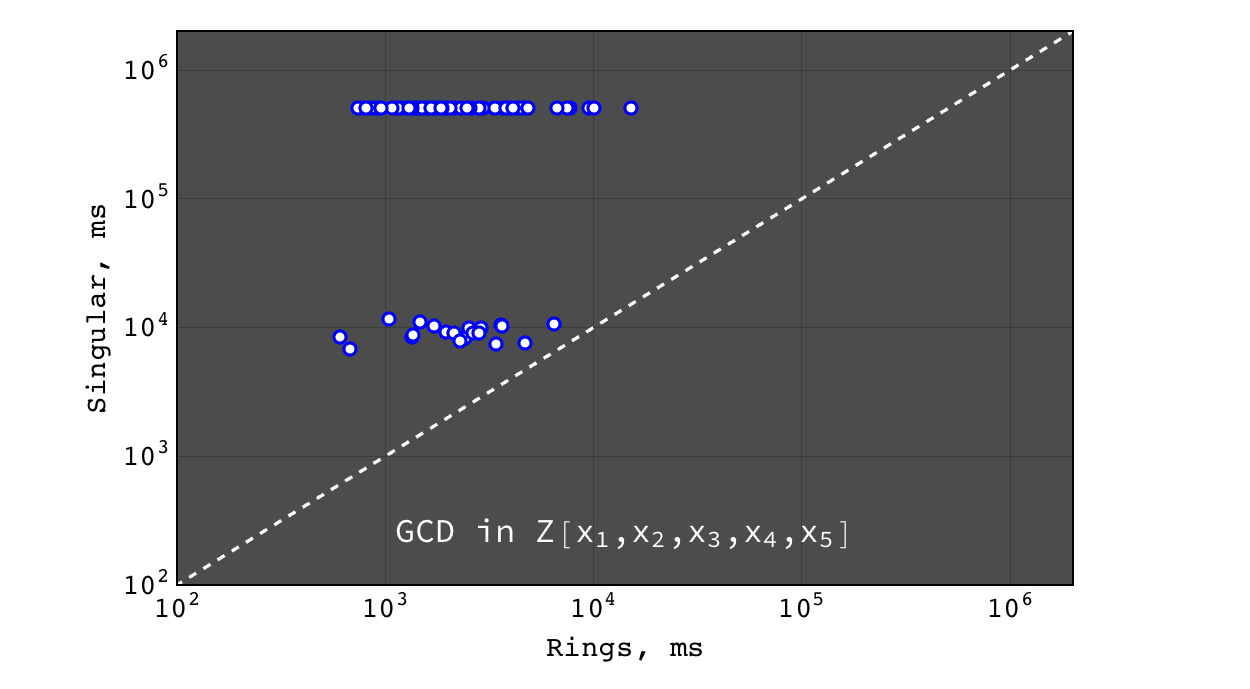Rings vs Singular performance of $$gcd(a g, b g)$$ for random polynomials $$(a, b, g) \in Z[x_1,x_2,x_3,x_4,x_5]$$ each with 40 terms and degree 20 in each variable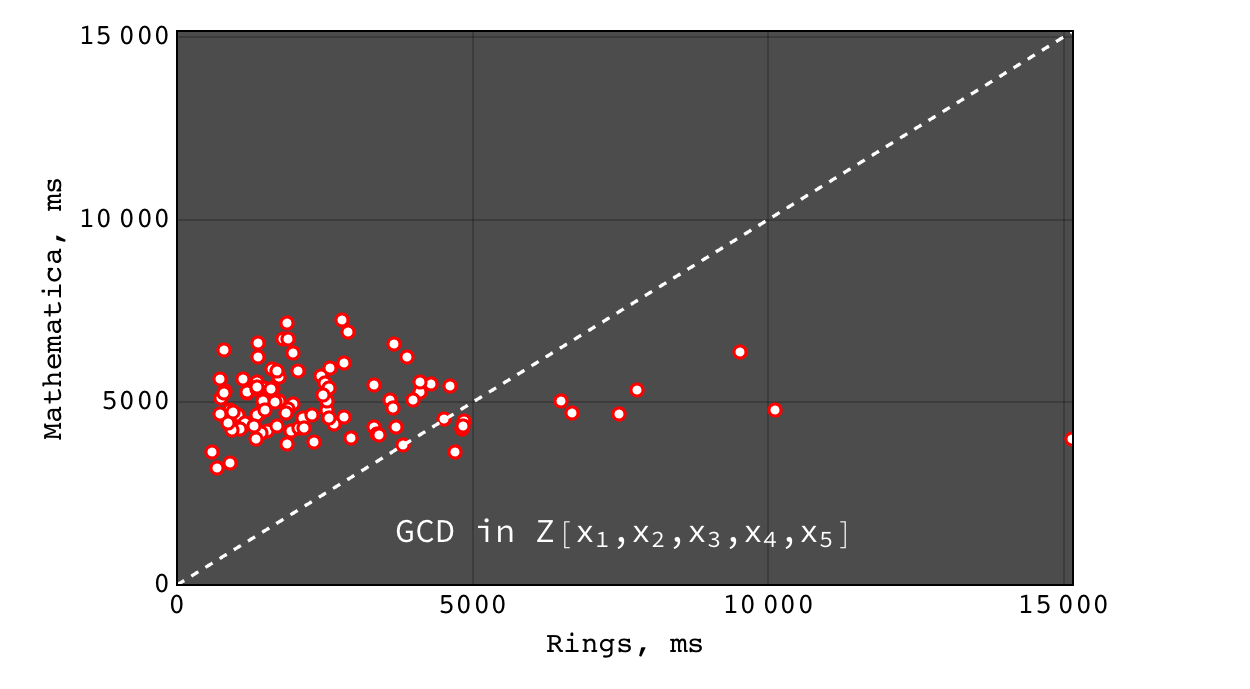Rings vs Mathematica performance of $$gcd(a g, b g)$$ for random polynomials $$(a, b, g) \in Z[x_1,x_2,x_3,x_4,x_5]$$ each with 40 terms and degree 20 in each variable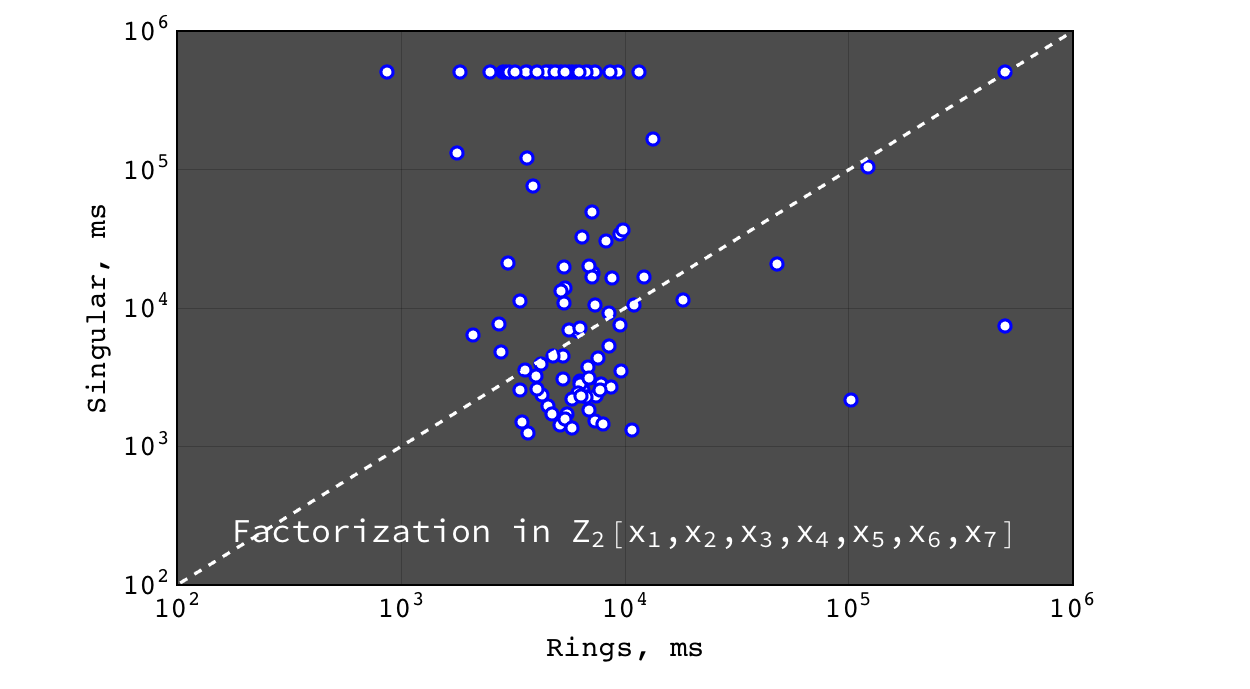Rings vs Singular performance of $$factor(a b c)$$ for random polynomials $$(a, b, c) \in Z_2[x_1,x_2,x_3,x_4,x_5,x_6,x_7]$$ each with 20 terms and degree 10 in each variable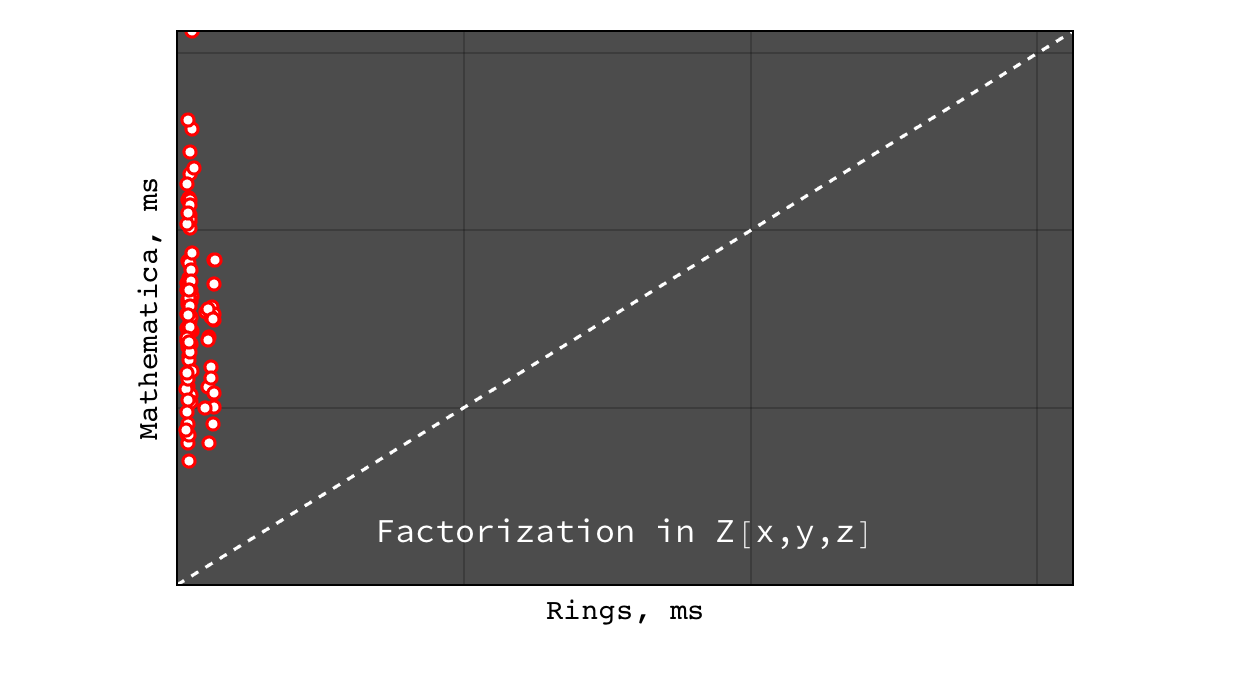Rings vs Mathematica performance of $$factor(a b c)$$ for random polynomials $$(a, b, c) \in Z[x,y,z]$$ each with 20 terms and degree 10 in each variable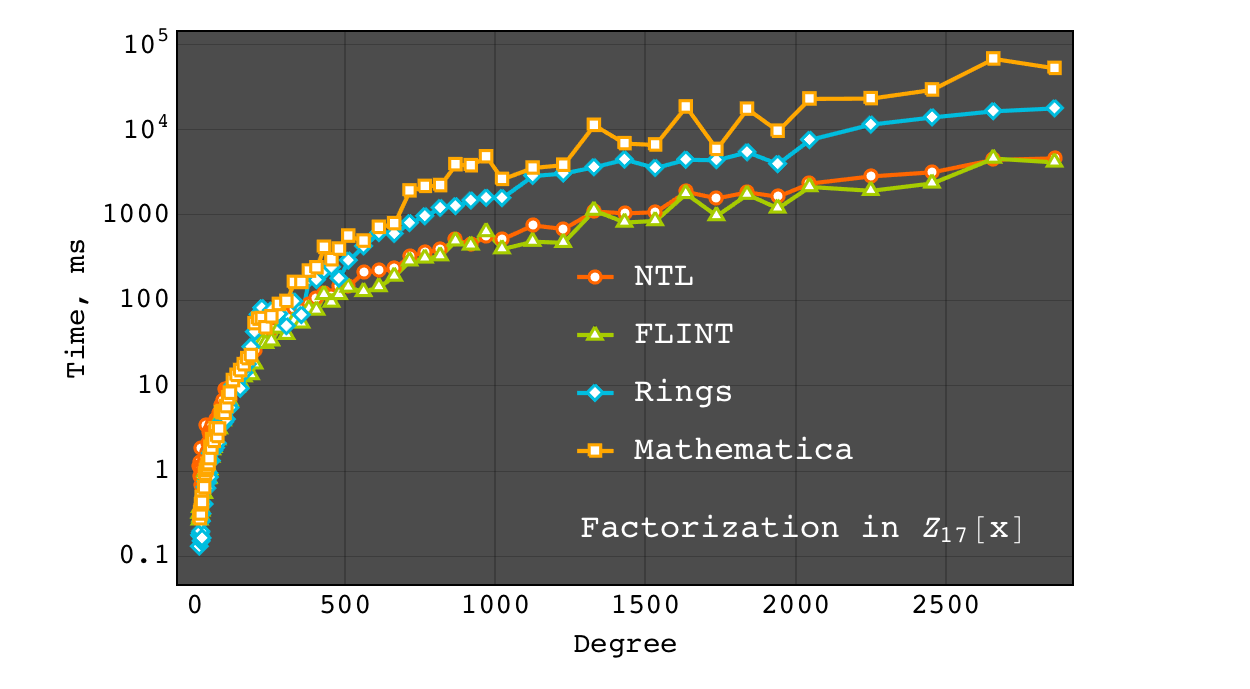Univariate factorization performance on polynomials of the form $$(1 + \sum_{i = 1}^{i \leq deg} i \times x^i)$$ in $$Z_{17}[x]$$. At small degrees the performance is identical, while at large degrees NTL and FLINT have much better asymptotic (probably due to more advanced algorithms for polynomial multiplication).